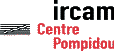Serveur © IRCAM - CENTRE POMPIDOU 1996-2005.Tous droits réservés pour tous pays. All rights reserved.

# Bifurcation, Period Doublings and Chaos in Clarinetlike Systems

C. Maganza, R. Caussé, F. Laloë

Europhysics Letters 1 (6), pp. 295-302 (1986)
Copyright © Europhysics Letters 1986

## Abstract

Wind instrument provide interesting hydrodynamical systems where non-linearities are important but well localized. A simple analysis shows that these systems should undergo Feigenbaum-type route to chaos, with a cascade of period doublings. Experiments have been performed with an acoustical resonator and an "artificial" excitation (nonlinearities controlled by either analogic or digital devices); they have confirmed these predictions.

Many musical instruments are in essence nonlinear physical systems, since their role is to convert a (quasi) constant force, pressure, etc. applied by the player into an oscillatory sound wave. For a physicist, the study of wind instruments is actually nothing but a subfield of hydrodynamics, since it amounts to studying the motion of the air inside and outside the instrument; the vibrations of the walls of the acoustical cavity are known to play a negligible role in the direct radiation of sound in the air, at least for woodwind instruments . Important progress has been made recently on nonlinear hydrodynamics, instabilities, turbulence, etc. (for a review, see for example ref  or ) and one could wonder how these ideas could apply to the study of musical instruments. One specific property of wind instruments is that the nonlinearities are indeed important, but well localized-mostly in the excitation system [4, 5]; this contrasts with ordinary hydrodynamics where they are present at every point in the fluid. The aim of the present work is to study how these localized nonlinearities can drive instabilities and chaos. A related work on acoustical chaos, including sound propagation but not acoustical resonators, is described in ref. .

The physical study of musical instruments is not new and much basic work was already done in the last century [7, 8] or 50 years ago . More recently, a nunber of advances in our understanding of wind instruments have occurred; for general reviews, see for example ref. ,  or . Examples of important new results are the studies of the role of the cut- off frequency of hole lattices [4, 11], and of good impedance peak co-operation [4, 12]. Here, we take a model of the functioning of a woodwind instrument which does not necessarily include all these physical effects, but gives the simplest description of nonlinear generation of sound from a constant pressure. The model we use draws heavily from the work published in ref  and  (sections II-B and II-D); see in particular the short discussion of period doubling in appendix A of ref . Among the instruments, we choose to concentrate on the clarinet, because it is simpler in many respects: its acoustical cavity i approximately cylindrical and the excitation mechanism uses a simple reed; double reeds for the oboe or bassoon, or flutelike excitation mechanisms are intrinsically more complicated. A clarinet reed is merely a thin, almost flat, flexible wedge of cane which moves under the effect of the pressures difference applied on both its sides (it tends to "close" or "open"); this changes the coupling between the applied pressure P0 inside the mouth of the player and the pressure p inside the acoustical resonator. A simple way to characterize the reed is to use a nonlinear function, f, which gives the flow of air entering the clarinet as a function of the pressure differencep = P0 - p; see fig. 1a). For a detailed discussion of the shape of the curve which gives f as a function ofp, the reader is referred for instance to  and . We simply note here that this curve includes negative (differential) impedance parts; as is well known in electronics for example, such negative impedances are required to sustain permanent oscillations.

In the model, we include only two variables, the pressure p and the flow f, which are both functions of time t; more precisely, we define f as the flow divided by the cross-section of the cylindrical resonator (f then gives the air velocity). Since we ignore the dynamics of the reed, we simply write as a first equation

f(t) = F[p(t); P0]

(1)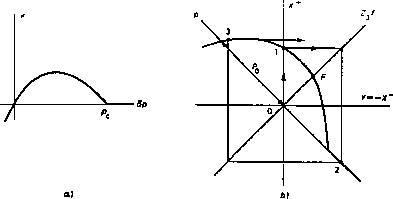Fig. 1. - a) Nonlinear characteristics of the excitation system, giving the air flow f as a function of the pressure differencep = P0 - p across the reed. Whenp = pc the reed closes and f vanishes. b) Geometrical construction giving the successive values ofp and f, obtained by a nonlinear iteration using the function of a) after translation and a 45º rotation. O, 1, 2, 3 are successive iterates. F is an (unstable) fixed point. The case shown corresponds to no dissipation (= o).
The second equation is obtained by writing a boundary condition which expresses the fact that the acoustical wave is a purely outgoing wave from the instrument (energy flows out from the bell and the open holes). If f(cL) and r() are the Fourier transforrn off(t) and p(t), this gives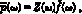where Z(w) is the acoustical impedance of the resonator measured at a point close to the reed. If G(t) is the inverse Fourier transform of Z(w), one then obtains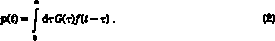Equations (l) and (2) provide a closed system: (1) is nonlinear, but local in time; on the other hand, (2) is linear but includes multiple time delays, as we now discuss.

An idealized resonator  can be obtained by considering a tube with constant section ending on a small acoustical impedanceZ0, assumed to be real, positive, and frequency independent (Z0 =c is the acoustical impedance in the open air). If any other source of dissipation (viscosity, heat conduction) is ignored, one easily obtainswhereis the Dirac peak function, and T= 2L/c is the time taken by a sound wave to travel twice the length L of the tube. Clearly, this form of G(t) implies multiple delays, and complicates the solution of the problem. As discussed in  and , an elegant way to avoid this difficulty is to introduce two new variables X+ and X-, the amplitudes of the outgoing and ingoing waves: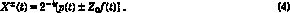Equation (2) then becomes: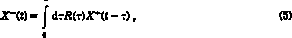where R() is the so-called "reflexion function"; its Fourier transform R(w) is obtained from Z(w) by the homographic transformation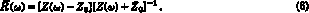For the idealized resonator considered above, eq. (3) leads to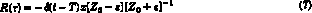with only one time delay.
The convenient variables are, therefore, X+ and X- rather than p and f. Equation (1) can be revwritten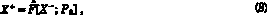where the graph of F is obtained from that of F (with variables p and Z0f) by a 45º rotation around the origin. Now, if we set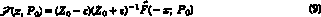and make use of (7), we obtain the final equation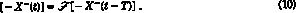The approximations that we have made lead to a particularly simple result: the time dependence of the variable Y = - X-(t) is given by an iteration with the F function. We shall discuss here only particular solutions (it is easy to generalize), steplike solutions which remain constant and equal to Yn in any time interval nT < t < (n + 1) T, and then jump to the next value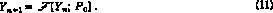The corresponding geometrical construction is shown in fig. lb). When the time dependence of X- is obtained, equation (8) gives X+ and (4) allows one to come back to p and f. The time variations obtained in this way are similar to those shown in fig. 5 of ref. .

Iterations and Feigenbaum-type scenarios  appear often in the study of strongly dissipative systems . This is not the case here, sinceis usually small (typically less than 0.1) and can even be put to zero.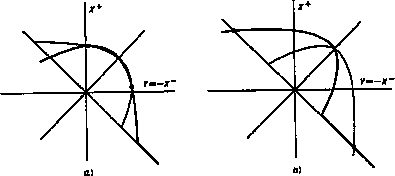Fig. 2. - Geometrical construction of the permanent oscillation values just above threshold, obtained by intersecting the characteristic curve with its symmetric with respect to the f-axis. In fig. a), small oscillations are obtained just above threshold, but not in fig. b)
Another difference with the usual case is that the "control parameter" P0 does not correspond to a "gain" on the nonlinear curve, but to a translation along a line at 45º to the axes. For small values of P0, the fixed point (intersection of the 45º line with the curve) i stable, since it has a slope between -1 and + 1: no oscillation occurs. When it reache the maximum of the p-f curve of fig. 1a) (that is when the differential impedance changes sign), the fixed point F become unstable: the clarinet starts to sound. The two values between which Y oscillates are obtained by intersecting the p-f curve with its symmetric with repect to the f-axes. If the curve has a steeper slope in the small pressure difference region, as in fig. 2a), small oscillations between almost equal values of Y are obtained just above threshold. On the other hand, if the opposite occurs, the bifurcation takes a different character, since the system jumps suddenly to a large level of oscillation (fig. 2b)). Fortunately, as noted by the authors of ref. , the f curve of real clarinets belongs to the first class of asymrnetry , which ensures the possibility for small oscillations and pianissirno playing.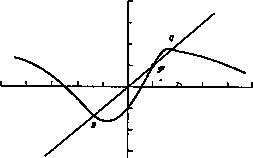Fig. 3. - The second iterate of F;Q and R give the permanent regime of normal oscillation, until they become unstable (slope less than - l); then, period doubling occurs.
It is well known  that the stability of a 2-cycle of oscillations can conveniently be discussed in terms of the second iterate of F for which the 2-cycle corresponds to two stable fixed points Q and R shown in fig. 3. When the slope at these points exceeds 1 (in absolute value), the cycle becomes unstable and gives rise to a 4-cycle: a period doubling occurs in the system. Period doubling should, therefore, in principle be observable in clarinet-like systems. Nevertheless, in practice, with f-p characteristics similar to that of fig. 1a), our numerical calculations have shown that period doubling can only be obtained in a rather narrow range of the parameters, which might explain why the phenomenon does not seem to be known among real clarinet players.

To do an experiment, it was, therefore, natural to try to obtain more flexibility on the nonlinearity introduced by the acoustical excitation system. The principle involved is shown in fig. 4; an ordinary acoustical resonator was used, in several cases a real clarinet, but the nonlinear reed excitation was replaced by an electroacoustic equivalent: a microphone measuring the acoustical pressure p inside the tube, a nonlinear system to include controlled nonlinearities in the feedback loop, and finally a loudspeaker to create a p-dependent air velocity f. The nonlinear system was either an analog electronic device, as in ref. , or an included digital processing of the data with a computer. The latter case gives even more flexibility on the choice of the nonlinear function, but requires using analog-digital converters, which introduces time delays (of the order of 100 µs in our experiment with two converters and the 4C computer of the IRCAM).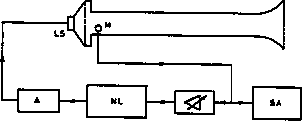Fig. 4. - Sketch of the experimental set-up. The reaction loop includes a microphone M, a variable gain amplifier, a nonlinear system NL (either an analogic circuit or a digital device including AD converters and a computer), a power amplifier A and finally a loudspeaker LS. The signals are analysed through a spectrum analyser SA.
Figure 5 shows the results obtained in an experiment where the nonlinearity was obtained with an analog electronic circuit generating the function x3 - x; the resonator was simply a plastic tube (length: 14 cm; inner diameter: 2 cm) The results shown in fig. 5 are the Fourier spectra provided by the spectrum analyser; they correspond to increasing values of the gain in the nonlinear reaction loop. A series of three successive period doublings was obtained, the last one resulting in a very low pitch sound (70 Hz), which is very unusual with such a short resonator tube. All the period doublings could be clearly heard as sounds jumping one octave below, superimposed on some random acoustical noise, especially for the third bifurcation which is rather unstable. Beyond this bifurcation, acoustical chaos could be seen on the spectrum analyser and heard in the laboratory.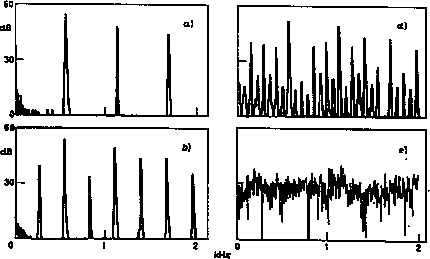Fig. 5. - Results of our experiments showing the spectra obtained on the SA for increasing values of the gain in the feedback loop. a) Normal oscillation; b), c) (not shown) and d) are, respectively, the first, second and third period doublings; e) chaotic regime.
The number of observed period doublings might have been limited by the narrow domain of the experimental parameters in which they occur, but also by the poor efficiency of the electroacoustic components at low frequencies. The actual experiment is of course significantly different from an ideal experiment; for example phase rotations and band pass limitation are introduced by the loudspeaker. In practice, the time variations of the acoustical pressure have much smoother variations than steplike function. ALso, in the period doubling regime before the chaos limit, some random noise was already present, making the phenomenon slightly less clearly audible (and probably less interesting musically!).

Similar results were also obtained with digitally generated nonlinearities, including experiments with a real clarinet (with its mouthpiece removed). In the latter case, we never obtained more than two period doublings. This is not extremely surprising with a real clarinet, the bore is not cylindrical (later holes, bell, etc.), and the reflexion function cannot be well approximated by one delta-function. We were never able to observe any period tripling in any of the experimental conditions. A more detailed report on the experimental results obtained in various situations (e.g with different sorts of nonlinearities) will be published elsewhere. A natural prolongation of these experiments would be to use several acoustical resonators in parallel, to study for example the evolution from quasi-periodic to chaotic regimes, etc.

In conclusion, although our mathematical model and the geometrical construction used in fig. 1, 2 and 3 are limited by the various simplifications on which they rely, the experiments show that the essence of their predictions for various regims of oscillations remains valid in more realistic situations, including nonideal resonators such as a real clarinet. More generally, woodwind instruments belong to a class of nonlinear systems which exihibit interesting behaviour, especially if one allows for more flexible nonlinearities. It has been known for a long time  that woodwind instruments can generate harmonic frequencies which do not correspond to any resonance of the resonator (e.g the even harmonics in a clarinet tone; the effect is simply a frequency doubling occuring in the nonlinear excitation). The effects observed here show that even the fundamental tone of the generated sound can also, fall at a frequency which is much below any resonance of the tube.

The authors are grateful to J. KERGOMARD and G. WEINREICH for many stimulating discussions and helpful advices.

## References


J. BACKUS: J. Acoust. Soc. Am., 36, 1881 (1964); J. W. COLTMAN: J. Acoust. Soc. Am., 49, 520 (1971).
(2]
J. GUCKENHEIMER and P. HOLMES: Appl. Math. Sci., Vol. 42 (Springer Verlag, Berlin, 1983).

P.BERGE, Y. POMEAU and C. VIDAL: L'ordre Dans le Chaos, (Hermann, Paris, 1985).

A. H. BENADE: Foundamentals of Musical Acoustics (Oxford University Press, 1976).

Small nonlinear effects can indeed occur also in the acoustical resonator; see for example A. H. BENADE, preprint.

M. KITANO T. YABUZAKI and T. OGAWA: Phys. Rev. Lett., 50, 713 (1983).

H. HELMHOLZ: On the Sensation of Tone (Dover, New York, N. Y., 1954).

LORD RAYLEIGH: Theory of Sound (Dover, New York, N. Y., 1945).

H. BOUASSE: Instrument à vent (Delagrave, Paris, 1929).

C. J. NEDERVEEN: Acoutical Apects of Woodwind Instruments (Frits nof, Amterdam, 1969).

H. BENADE: J. Acout. Soc. Am., 32, 1591 (1960).

H. BENADE and D. J. GANS: N. Y. Acad. Sci., 155, 247 (1968).

R. T. SCHUMACHER: Acoustica, 48, 72 (1981).

M. E. MCINTYRE, R. T. SCHUMACHER and J. WOODHOUSE: J. Acout. Soc. Am., 74, 1325 (1983).

M. J. FEIGENBAUM Physica D, 7, 16 (1983).

## Notes


For a real resonator, the losses are introduced by gas viscosity and conduction, and not localized at the end of the tube;can be seen as a mere (positive) convergence factor, useful to take the limit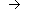0+. Also, for real resonators, there are frequency-dependent end corrections, the bore diameter is not necessarily exactly constant, etc., so that the reflexion function is not a-function; nevetheless, it has a significantly shorter memory than G(); see examples in .

____________________________
Server © IRCAM-CGP, 1996-2008 - file updated on .

____________________________
Serveur © IRCAM-CGP, 1996-2008 - document mis à jour le .